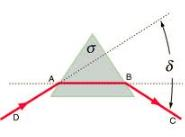Courses

# Test: Refraction And Dispersion By Prism

## 10 Questions MCQ Test Physics Class 12 | Test: Refraction And Dispersion By Prism

Description
This mock test of Test: Refraction And Dispersion By Prism for JEE helps you for every JEE entrance exam. This contains 10 Multiple Choice Questions for JEE Test: Refraction And Dispersion By Prism (mcq) to study with solutions a complete question bank. The solved questions answers in this Test: Refraction And Dispersion By Prism quiz give you a good mix of easy questions and tough questions. JEE students definitely take this Test: Refraction And Dispersion By Prism exercise for a better result in the exam. You can find other Test: Refraction And Dispersion By Prism extra questions, long questions & short questions for JEE on EduRev as well by searching above.
QUESTION: 1

### A mark on the bottom of the liquid appears to rise by 0.1 m. The depth of the liquid is 1 m. The refractive index of the liquid is​

Solution:

As per the given condition,
Real depth of the liquid= 1 m
Apparent depth of the liquid =1−0.1=0.9 m
Refractive index of the liquid = Real Depth/Apparent Depth ​=1/0.9​=1.11

QUESTION: 2

### Dispersive power is dependent on

Solution:

Dispersive power depends on the material of the prism. When light transitions from one medium to another it bends on the basis of the refractive indices of the two media. Dispersive power is a measure of the amount of difference in the refraction of the highest and lowest wavelengths which enter the prism.

QUESTION: 3

### Waves on top of spectrum are

Solution:

Infrared: Night vision goggles pick up the infrared light emitted by our skin and objects with heat. In space, infrared light helps us map the dust between stars.
Visible: Our eyes detect visible light. Fireflies, light bulbs, and stars all emit visible light.

Ultraviolet: Ultraviolet radiation is emitted by the Sun and are the reason skin tans and burns. "Hot" objects in space emit UV radiation as well.

X-ray: A dentist uses X-rays to image your teeth, and airport security uses them to see through your bag. Hot gases in the Universe also emit X-rays.

Gamma ray: Doctors use gamma-ray imaging to see inside your body. The biggest gamma-ray generator of all is the Universe.

QUESTION: 4

Which is the position of minimum deviation in prism?

Solution:

The angle of deviation is minimum when the path of light ray through the prism is symmetrical. i.e., angle of incidence = angle of emergence and path of ray within the prism is parallel to the base of the prism.QUESTION: 5

The ratio of angular dispersion of to the angle of deviation for the mean wavelength is called Dispersive Power. Represented by

Solution:

The dispersive power(ω)Of a prism is defined as the ratio of angular dispersion to the mean deviation produced by the prism,
Mean deviation=��
Angular dispersion= ��v- ��y
Dispersive power=angular dispersion/mean deviation
Dispersive power= ��v- ��y/ ��

QUESTION: 6

Angle of dispersion depends on

Solution:

The dispersion is the difference in refracting of lights with highest and lowest velocities. Dispersion depends upon the refractive indexes of the two mediums. Thus, depending on the material of the prism.

QUESTION: 7

Which relation do we use to find the refractive index of material of prism

Solution:
QUESTION: 8

A prism of refractive index √2 and refractive angle A produces minimum deviation δm of a ray on one face at an angle of incidence 45°. The values of A and δm are respectively​

Solution:
QUESTION: 9

What is dispersive power?

Solution:

Dispersive power of a prism is defined as the ratio between angular dispersion to mean deviation produced by the prism.
If dμ denotes the difference between the refractive indices of material of prism for violet and red light,
ω = δμ / μ – 1
Here ‘μ’ is the refractive index of prism for a mean colour. A mean colour is that colour whose wavelength lies in between that of violet and red. For white light, yellow colour is, generally, taken to be the mean colour.
Since μv is always greater than μr, the dispersive power of a prism is always positive. It depends upon the type of glass used. It is different for crown glass and for flint glass.

QUESTION: 10

A thin prism P1 with angle 4° and made from glass of refractive index 1.54 is combined with another thin prism P2 made from glass of refractive index 1.72 to produce dispersion without deviation . The angle of prism P2 is:​

Solution:

For dispersion without deviation,
1​−1)×A1​=(μ2​−1)×A2​
μ1​=1.54, A1​=4°, μ2​=1.72
(1.54−1)×4=(1.72−1)×A2​
⇒ A2​=3